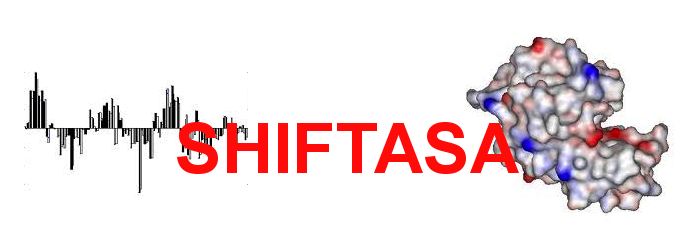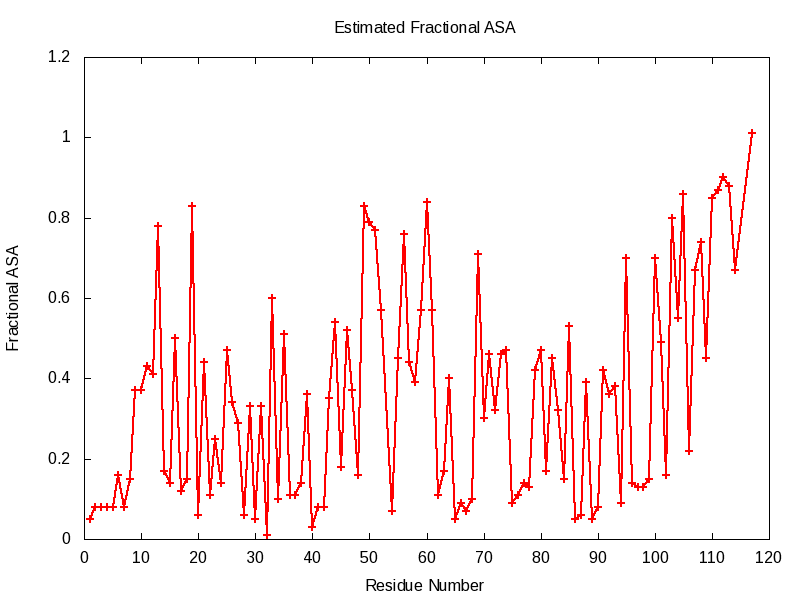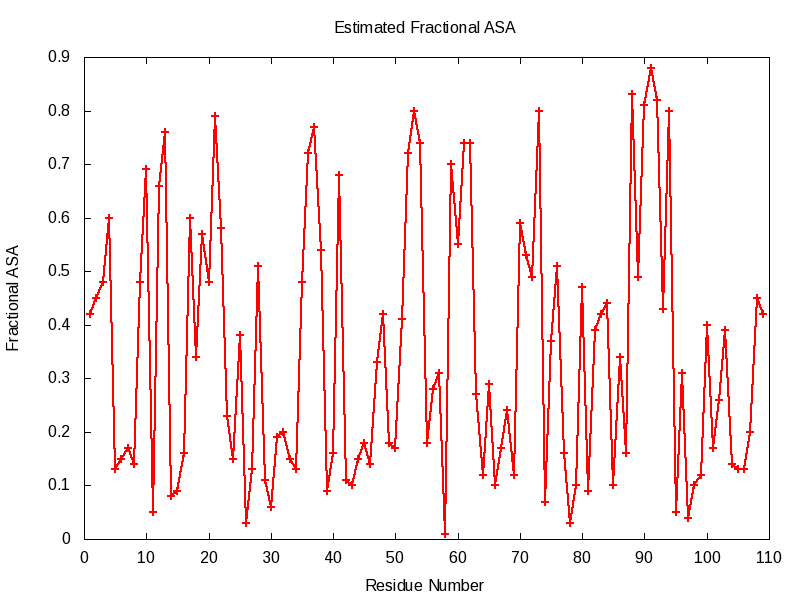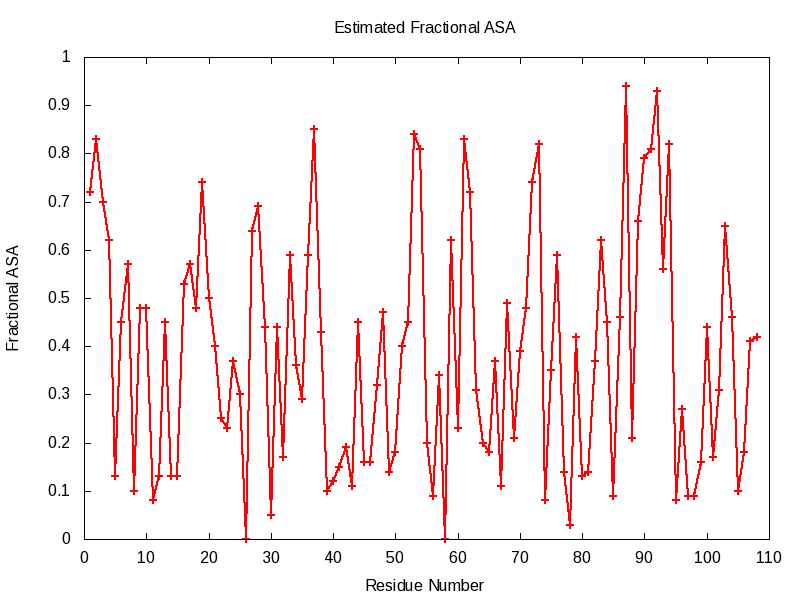PDB:1O13(A) BMRB:6198 Name:Dinitrogenase(Thermotoga Mortima) Estimated Fractional ASA Horizontal Output Vertical Output Num = Residue number Res = Residue name fASA = fractional ASA Cls = Buried/Exposed class based on 25% threshold Fractional ASA GraphPDB:1RWY(B) BMRB:15517 Name:Alpha-Parvalbumin(Rat) Estimated Fractional ASA Horizontal Output Vertical Output Num = Residue number Res = Residue name fASA = fractional ASA Cls = Buried/Exposed class based on 25% threshold Fractional ASA GraphPDB:1RRO(A) BMRB:7322 Name:Recombinant Oncomodulin Estimated Fractional ASA Horizontal Output Vertical Output Num = Residue number Res = Residue name fASA = fractional ASA Cls = Buried/Exposed class based on 25% threshold Fractional ASA Graph